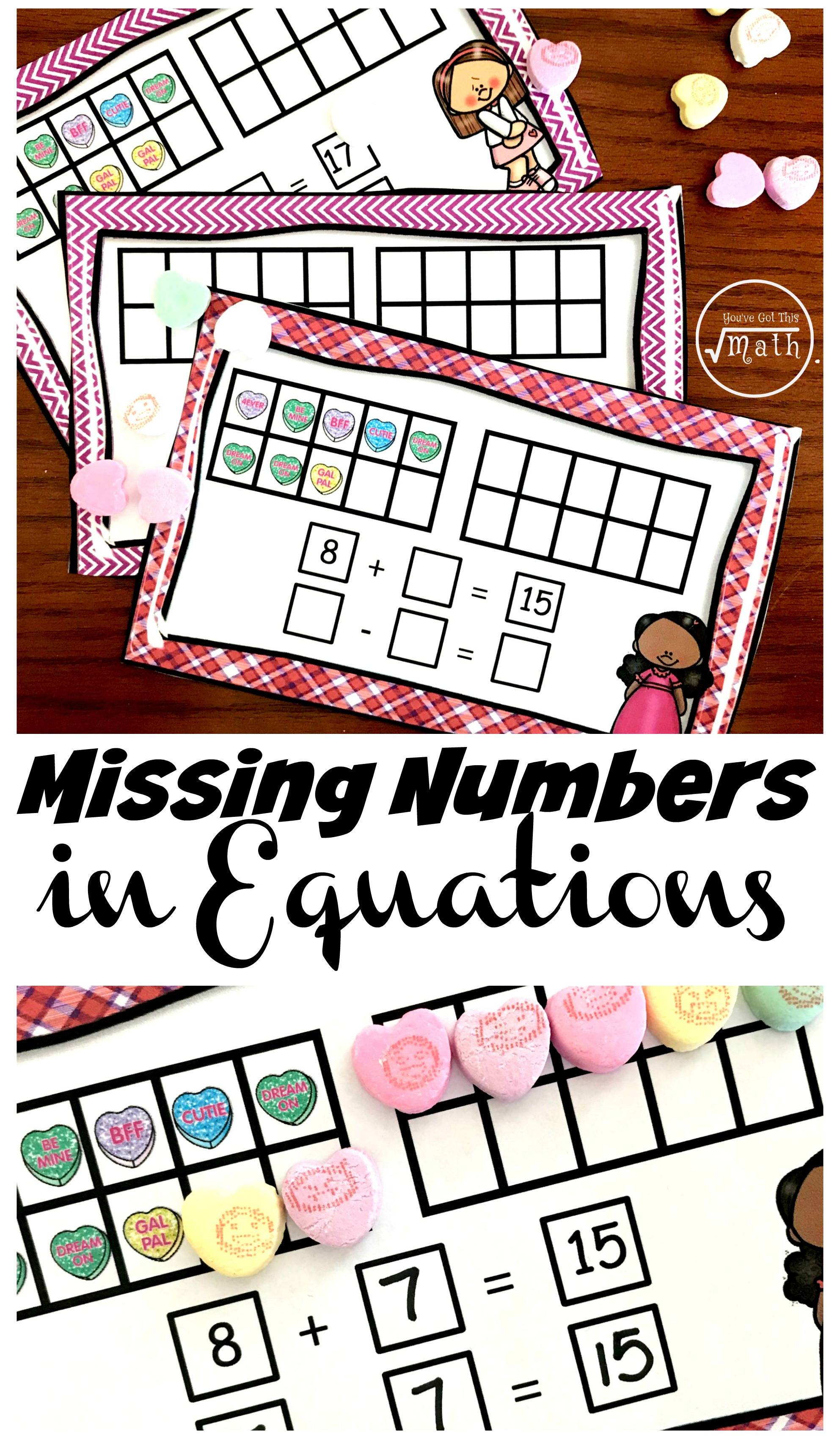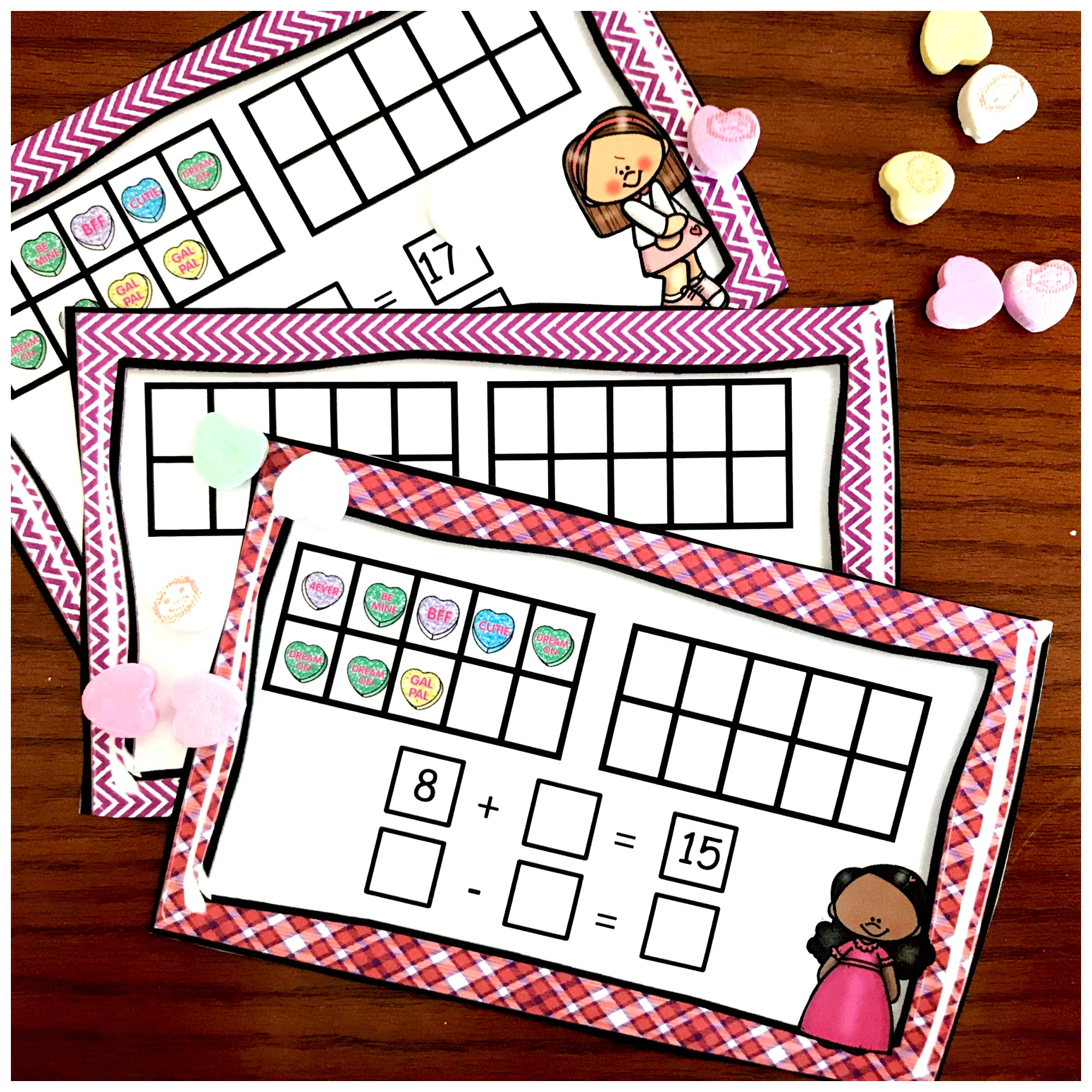# Fun, Hands-On Task Cards For Teaching Missing Numbers in Equations

These task cards are a great way to help children practice filling in missing numbers in equations. The ten frames and manipulatives help kiddos struggling in math to be successful.

Grab this free printable by clicking on the bluish button at the bottom of the post.

##My six-year-old just gets math! It is once in a blue moon, that I have to review a lesson or help him since he is struggling. I must admit I was surprised when finding a missing value in a number sentence was a little difficult for him at first.

Finding the missing numbers in equations is an important skill. It solidifies the understanding of fact families and builds an understanding that will help them conqueror fractions.

So I know that we will be spending a little more time on this concept with my first grader, making sure that this small struggle becomes a victory for him. And one way we are doing this is with hands-on task cards using conversation hearts.

## Prep -Work –

First, print off task cards on card stock paper.
Next, cut out and laminate.
Finally, provide dry erase markers and conversation hearts.

## Directions for Missing Numbers In Equations

So the directions are pretty simple, have your children use conversation hearts to fill in the 10 frames and find the missing number!

Here is one example!! This task card has the expression  8 + ___ = 15  As you can see, eight of the spaces on the ten frame are already filled in. Since the answer is 15, we need to have 15 hearts on the ten frame. So the student will add conversation hearts until there are a total of 15.

Once they have 15 hearts on the ten frames, it is time to figure out the answer. All our little ones need to do is count the amount of hearts they added. Then they can fill in the first blank.

8 + 7 = 15

Now it is time for the subtraction part. And this is just understanding fact families or fact triangles. If your students have practiced fact families they should know that all the related addition and subtraction for 8 + 7 = 15 are….

7 + 8 = 15

15 – 7 = 8

15 – 8 = 7

Now they just write one of the subtraction families into the blanks.

There are also some task cards that have children figuring out the missing number in equations that focus on subtraction. In this example, the children are not given the minuend, the part you normally would start with. What they are given is the subtrahend, the part being subtracted, and the difference.

We all have learned that when we add the subtrahend and the difference, we get the minuend. And that is all they need to do here. They start by placing down three hearts.

#### Subtraction Equations

Next, they add twelve hearts and count up the number of hearts in the 10 frames. As you can see from the example above, there are a total of 15 hearts. Therefore the answer is 15 – 3 = 12

This type of practice builds number sense for our little ones and prepares a strong basis for when they have to tackle algebra down the road.

I hope you enjoy exploring with your little ones.

You’ve Got This

Rachel

Missing-Numbers-in-Equations

You May Also Like:

This equation packet has five activities to work on equations. There are equation sorts, clip cards, task cards and more.